Скачать презентацию Geog 480 Principles of GIS Guofeng Cao Cyber

f8959342605197596caa30b7ff091131.ppt

• Количество слайдов: 72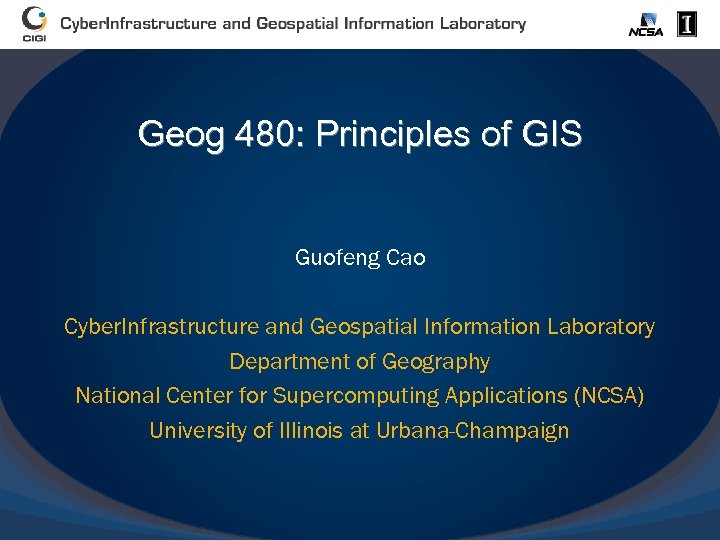Geog 480: Principles of GIS Guofeng Cao Cyber. Infrastructure and Geospatial Information Laboratory Department of Geography National Center for Supercomputing Applications (NCSA) University of Illinois at Urbana-Champaign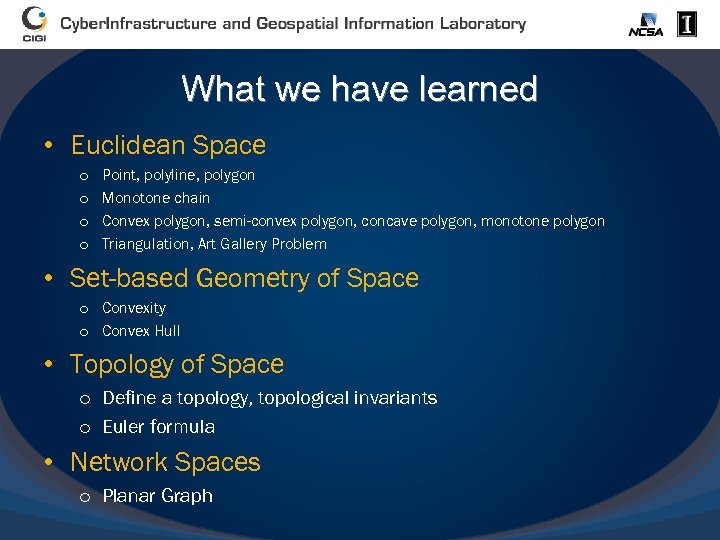What we have learned • Euclidean Space o o Point, polyline, polygon Monotone chain Convex polygon, semi-convex polygon, concave polygon, monotone polygon Triangulation, Art Gallery Problem • Set-based Geometry of Space o Convexity o Convex Hull • Topology of Space o Define a topology, topological invariants o Euler formula • Network Spaces o Planar Graph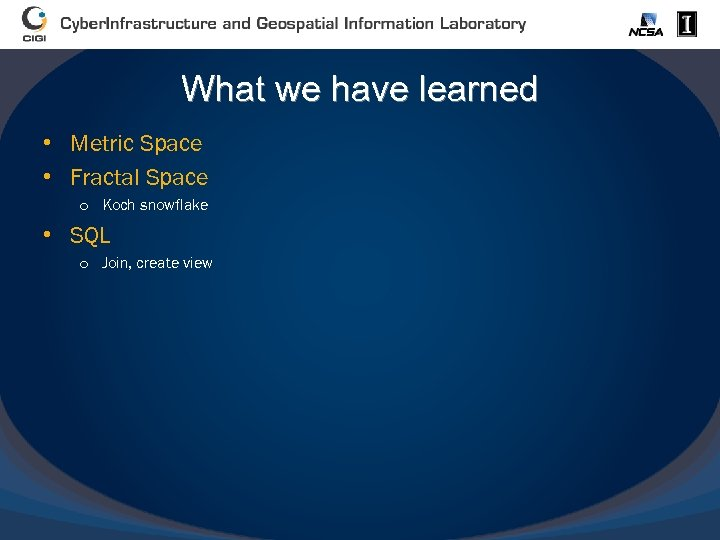What we have learned • Metric Space • Fractal Space o Koch snowflake • SQL o Join, create view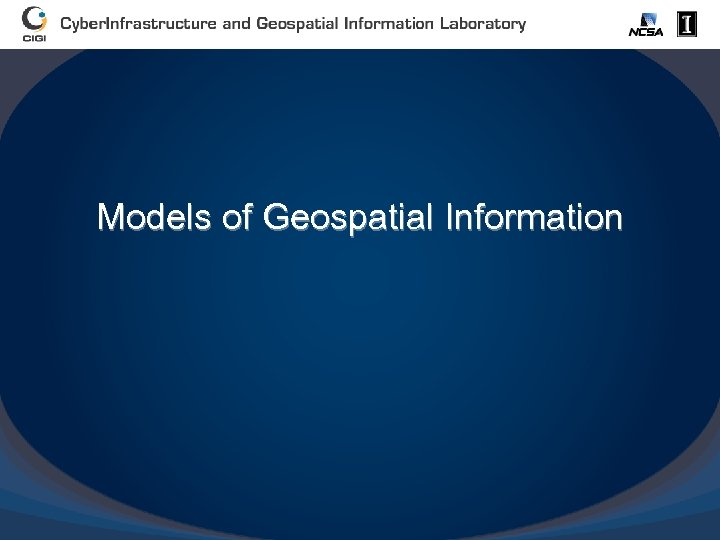Models of Geospatial Information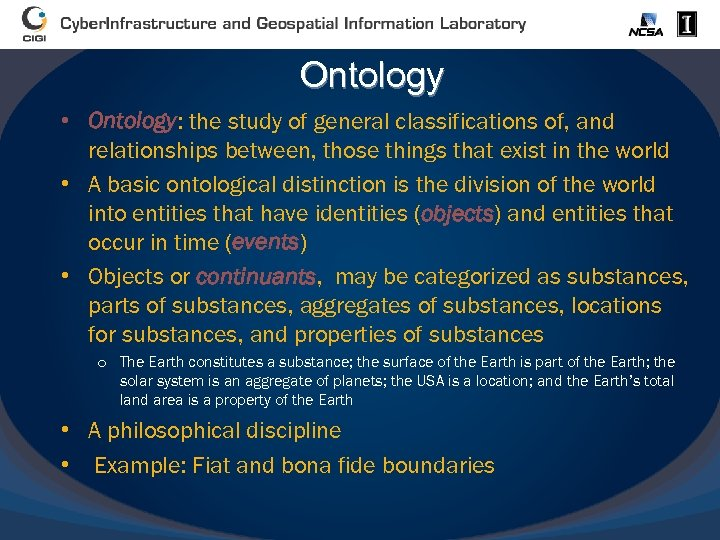Ontology • Ontology: the study of general classifications of, and relationships between, those things that exist in the world • A basic ontological distinction is the division of the world into entities that have identities (objects) and entities that occur in time (events) • Objects or continuants, may be categorized as substances, parts of substances, aggregates of substances, locations for substances, and properties of substances o The Earth constitutes a substance; the surface of the Earth is part of the Earth; the solar system is an aggregate of planets; the USA is a location; and the Earth’s total land area is a property of the Earth • A philosophical discipline • Example: Fiat and bona fide boundaries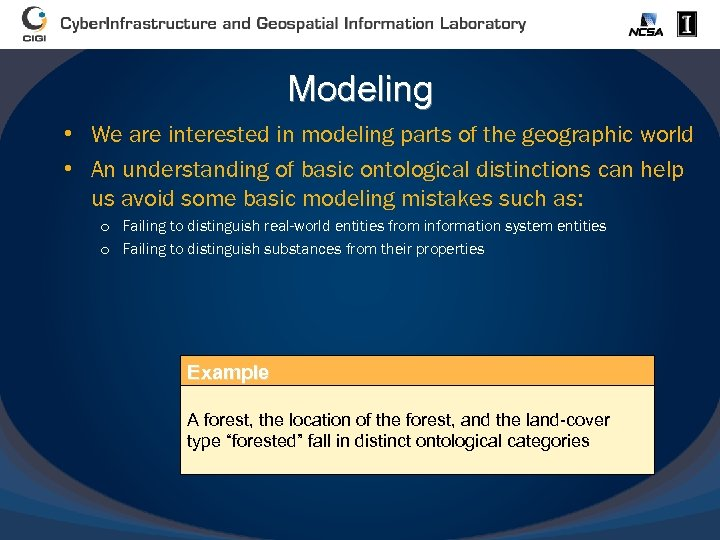Modeling • We are interested in modeling parts of the geographic world • An understanding of basic ontological distinctions can help us avoid some basic modeling mistakes such as: o Failing to distinguish real-world entities from information system entities o Failing to distinguish substances from their properties Example A forest, the location of the forest, and the land-cover type “forested” fall in distinct ontological categories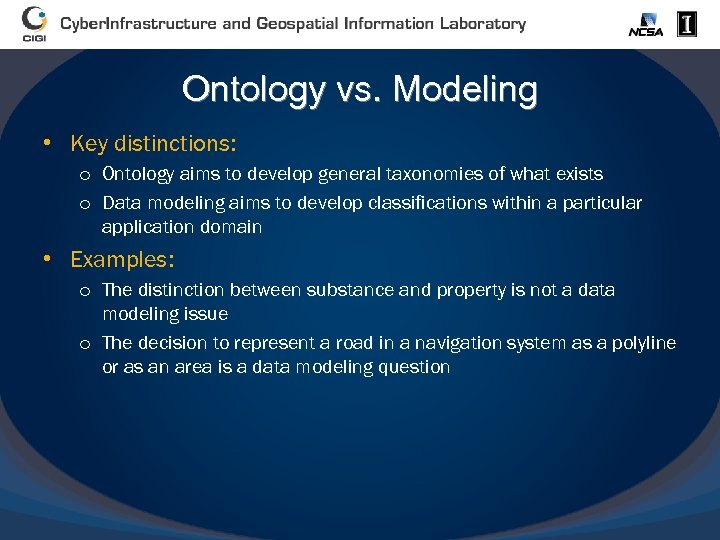Ontology vs. Modeling • Key distinctions: o Ontology aims to develop general taxonomies of what exists o Data modeling aims to develop classifications within a particular application domain • Examples: o The distinction between substance and property is not a data modeling issue o The decision to represent a road in a navigation system as a polyline or as an area is a data modeling question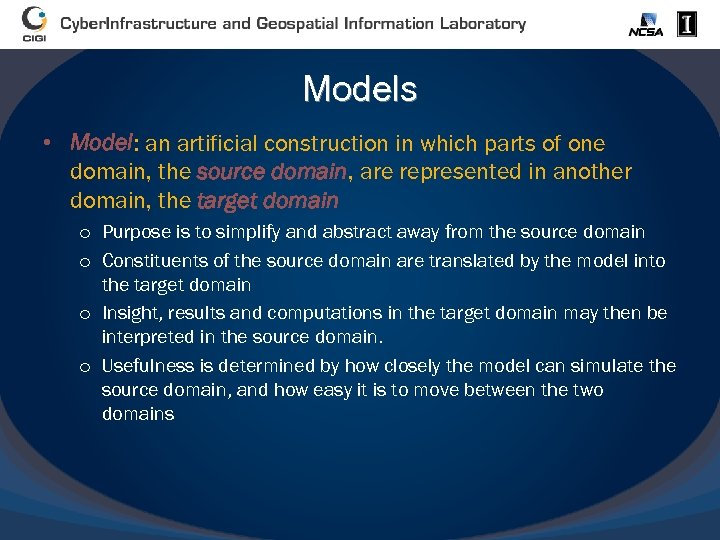Models • Model: an artificial construction in which parts of one domain, the source domain, are represented in another domain, the target domain o Purpose is to simplify and abstract away from the source domain o Constituents of the source domain are translated by the model into the target domain o Insight, results and computations in the target domain may then be interpreted in the source domain. o Usefulness is determined by how closely the model can simulate the source domain, and how easy it is to move between the two domains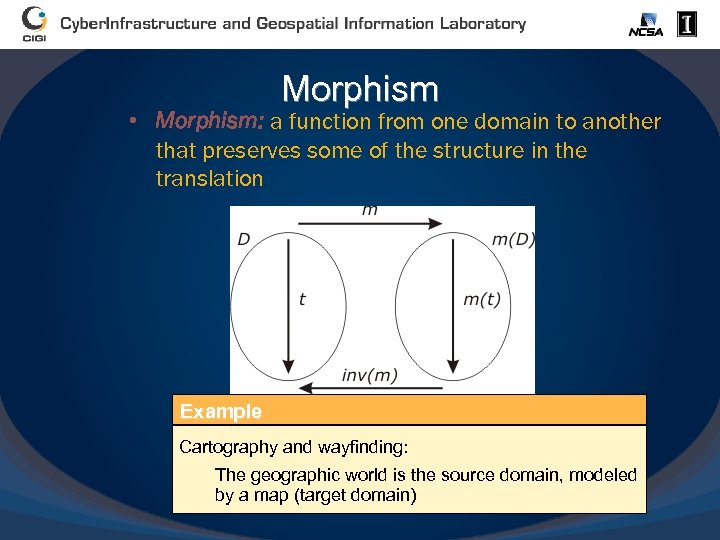Morphism • Morphism: a function from one domain to another that preserves some of the structure in the translation Example Cartography and wayfinding: The geographic world is the source domain, modeled by a map (target domain)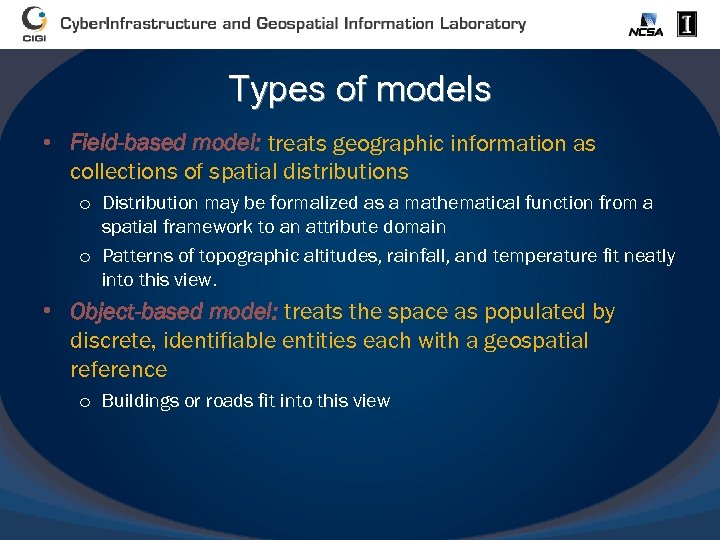Types of models • Field-based model: treats geographic information as collections of spatial distributions o Distribution may be formalized as a mathematical function from a spatial framework to an attribute domain o Patterns of topographic altitudes, rainfall, and temperature fit neatly into this view. • Object-based model: treats the space as populated by discrete, identifiable entities each with a geospatial reference o Buildings or roads fit into this view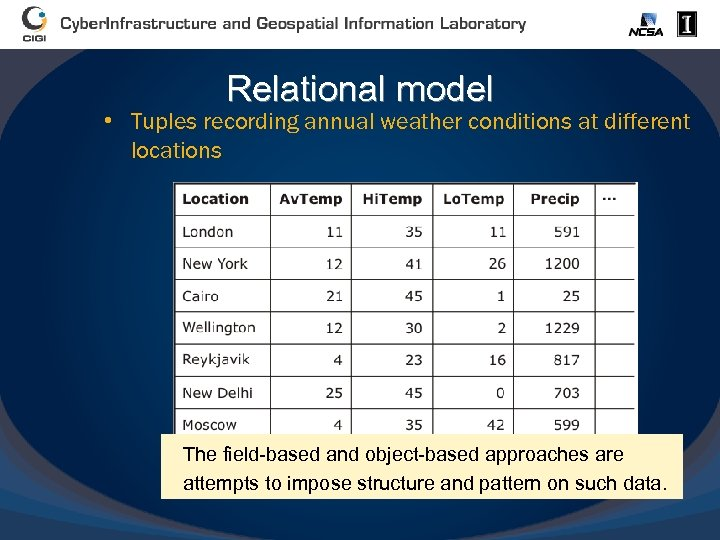Relational model • Tuples recording annual weather conditions at different locations The field-based and object-based approaches are attempts to impose structure and pattern on such data.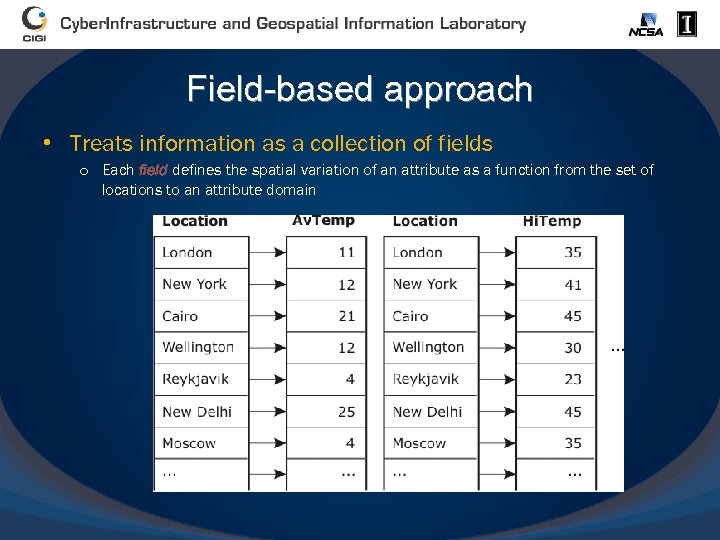Field-based approach • Treats information as a collection of fields o Each field defines the spatial variation of an attribute as a function from the set of locations to an attribute domain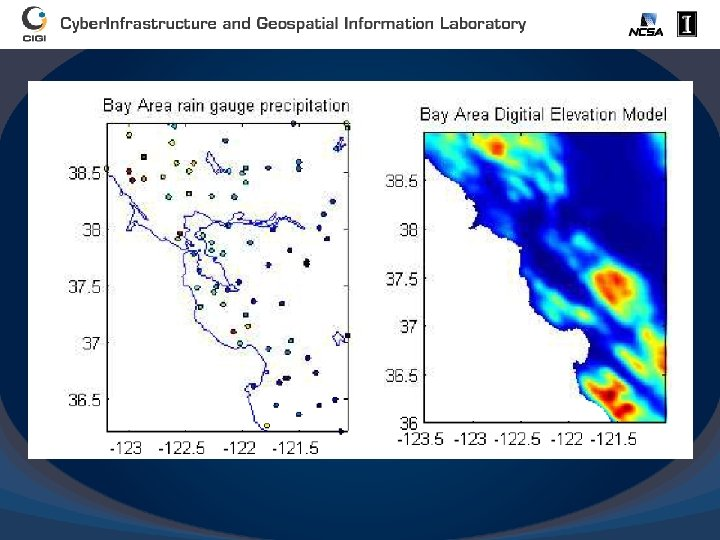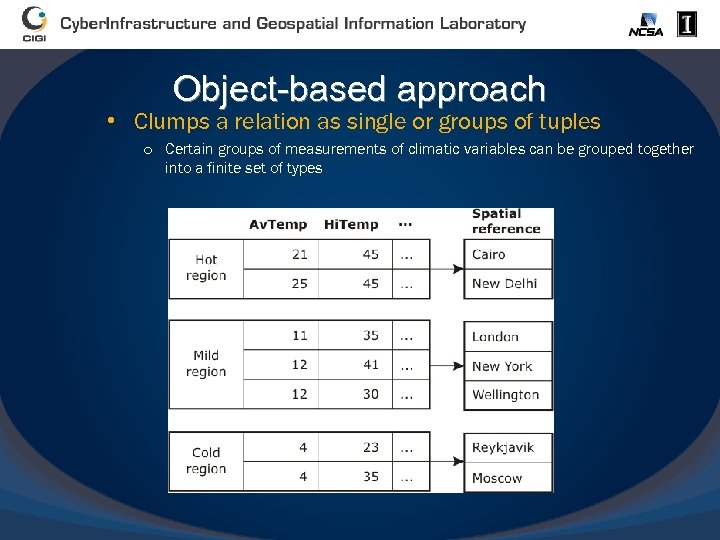Object-based approach • Clumps a relation as single or groups of tuples o Certain groups of measurements of climatic variables can be grouped together into a finite set of types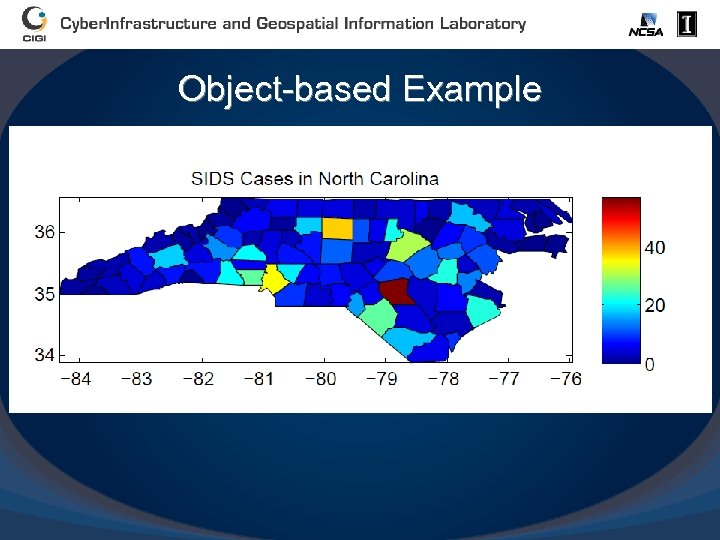Object-based Example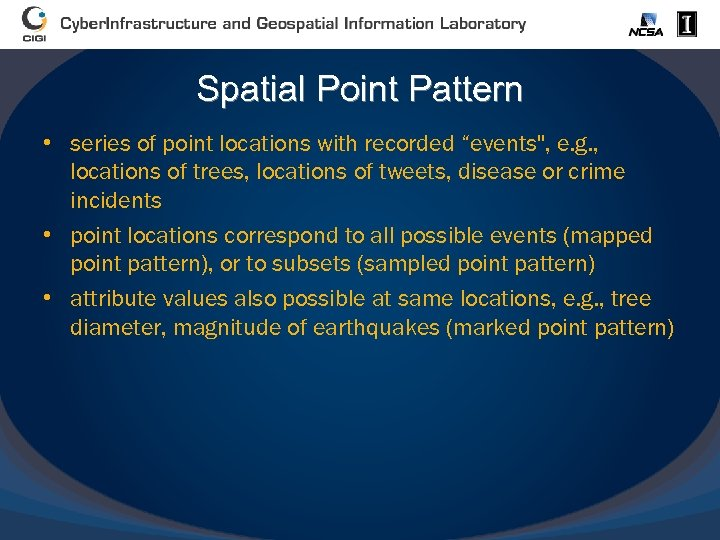Spatial Point Pattern • series of point locations with recorded “events", e. g. , locations of trees, locations of tweets, disease or crime incidents • point locations correspond to all possible events (mapped point pattern), or to subsets (sampled point pattern) • attribute values also possible at same locations, e. g. , tree diameter, magnitude of earthquakes (marked point pattern)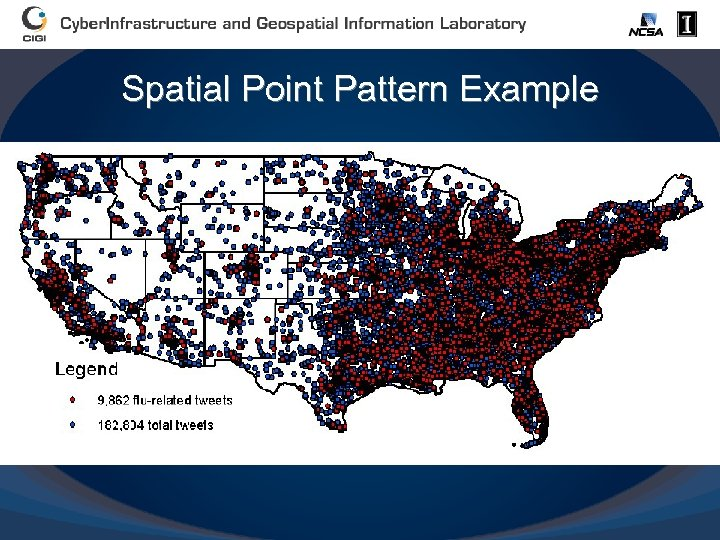Spatial Point Pattern Example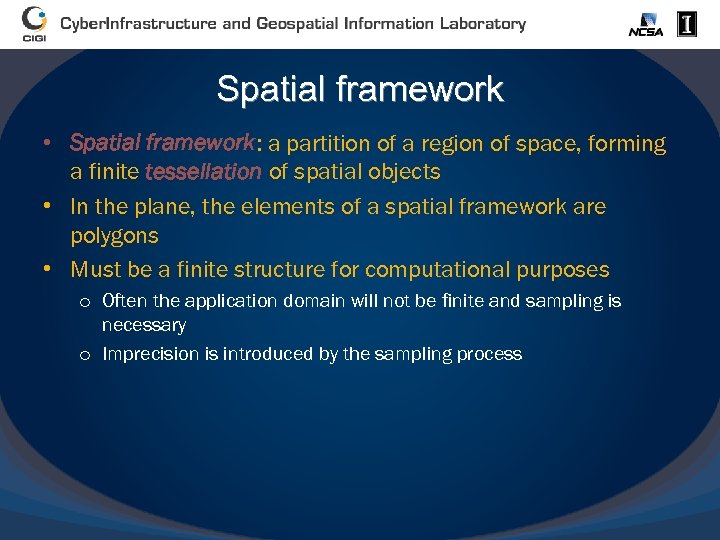Spatial framework • Spatial framework: a partition of a region of space, forming a finite tessellation of spatial objects • In the plane, the elements of a spatial framework are polygons • Must be a finite structure for computational purposes o Often the application domain will not be finite and sampling is necessary o Imprecision is introduced by the sampling process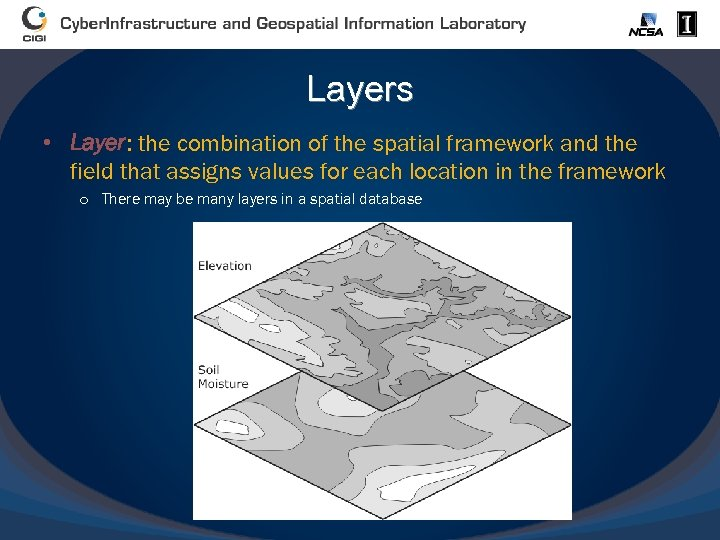Layers • Layer: the combination of the spatial framework and the field that assigns values for each location in the framework o There may be many layers in a spatial database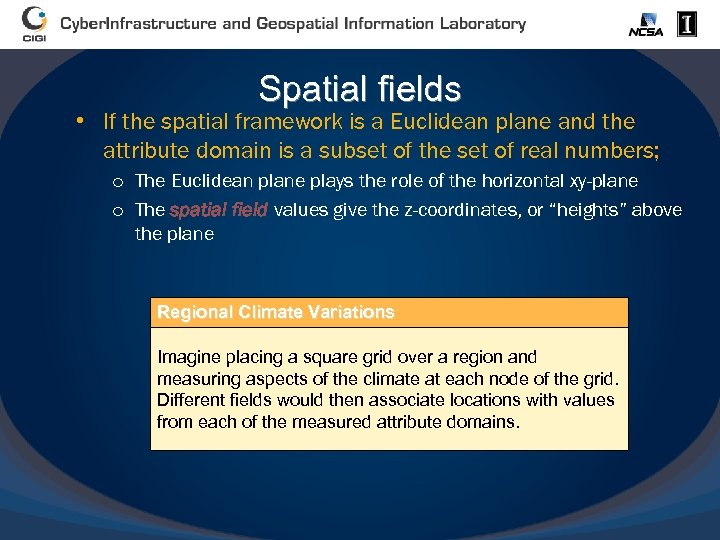Spatial fields • If the spatial framework is a Euclidean plane and the attribute domain is a subset of the set of real numbers; o The Euclidean plane plays the role of the horizontal xy-plane o The spatial field values give the z-coordinates, or “heights” above the plane Regional Climate Variations Imagine placing a square grid over a region and measuring aspects of the climate at each node of the grid. Different fields would then associate locations with values from each of the measured attribute domains.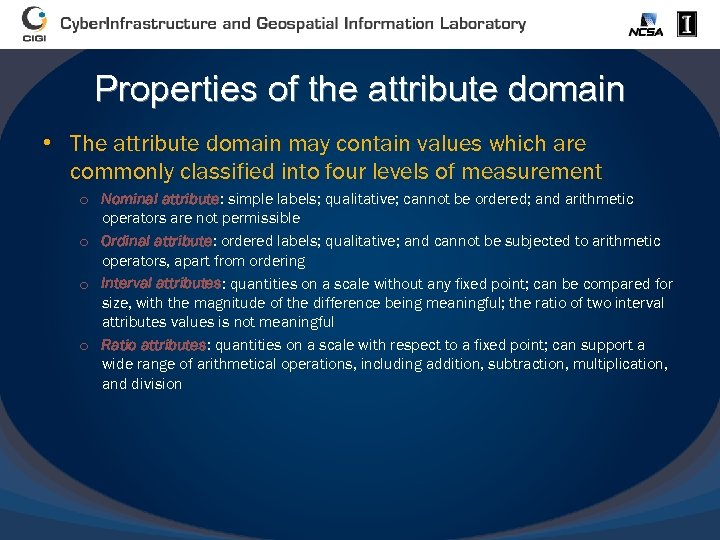Properties of the attribute domain • The attribute domain may contain values which are commonly classified into four levels of measurement o Nominal attribute: simple labels; qualitative; cannot be ordered; and arithmetic operators are not permissible o Ordinal attribute: ordered labels; qualitative; and cannot be subjected to arithmetic operators, apart from ordering o Interval attributes: quantities on a scale without any fixed point; can be compared for size, with the magnitude of the difference being meaningful; the ratio of two interval attributes values is not meaningful o Ratio attributes: quantities on a scale with respect to a fixed point; can support a wide range of arithmetical operations, including addition, subtraction, multiplication, and division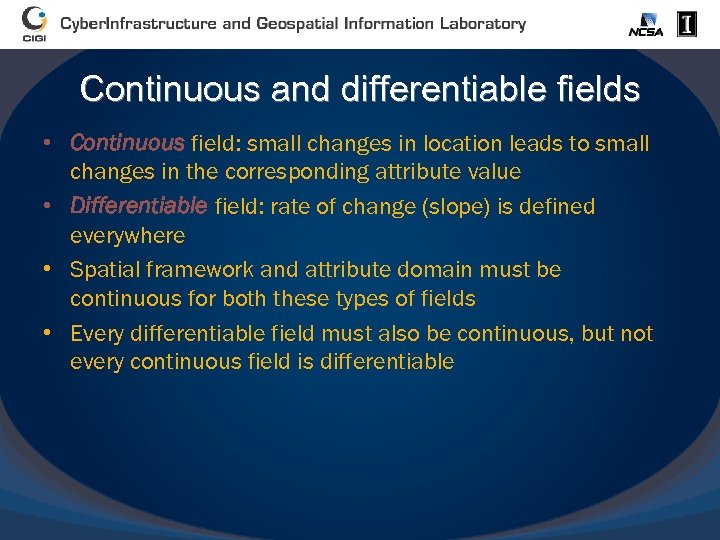Continuous and differentiable fields • Continuous field: small changes in location leads to small changes in the corresponding attribute value • Differentiable field: rate of change (slope) is defined everywhere • Spatial framework and attribute domain must be continuous for both these types of fields • Every differentiable field must also be continuous, but not every continuous field is differentiable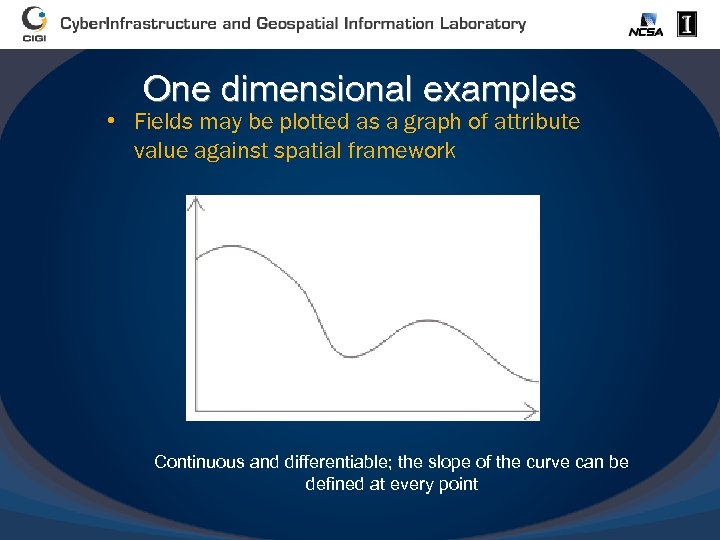One dimensional examples • Fields may be plotted as a graph of attribute value against spatial framework Continuous and differentiable; the slope of the curve can be defined at every point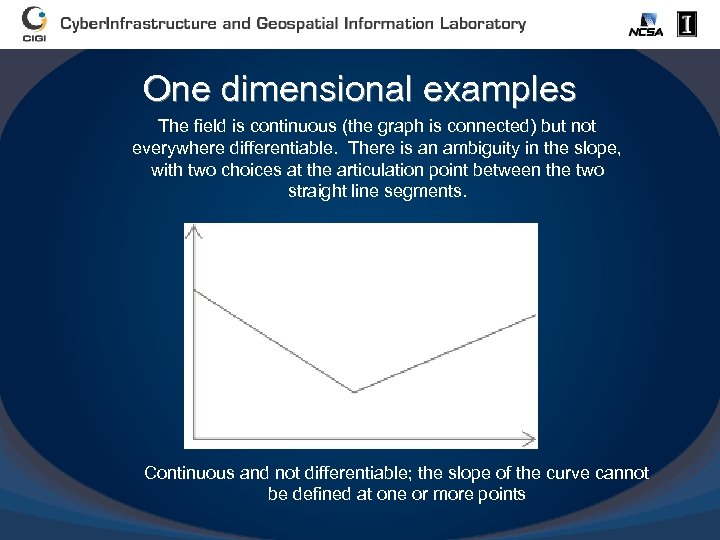One dimensional examples The field is continuous (the graph is connected) but not everywhere differentiable. There is an ambiguity in the slope, with two choices at the articulation point between the two straight line segments. Continuous and not differentiable; the slope of the curve cannot be defined at one or more points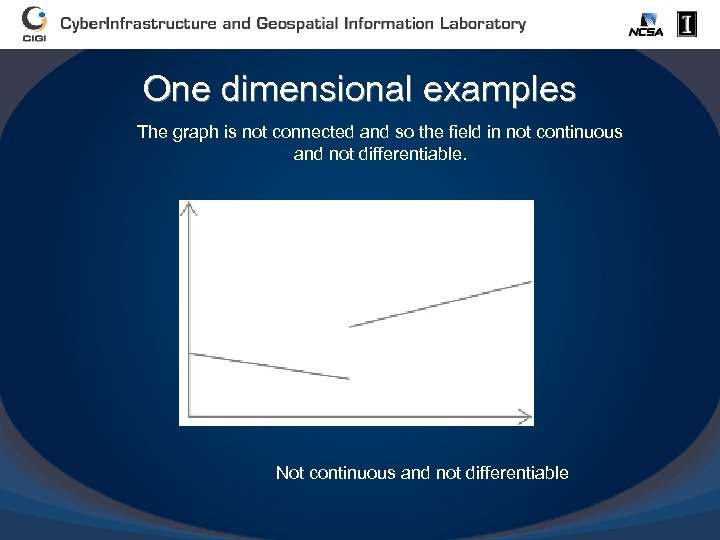One dimensional examples The graph is not connected and so the field in not continuous and not differentiable. Not continuous and not differentiable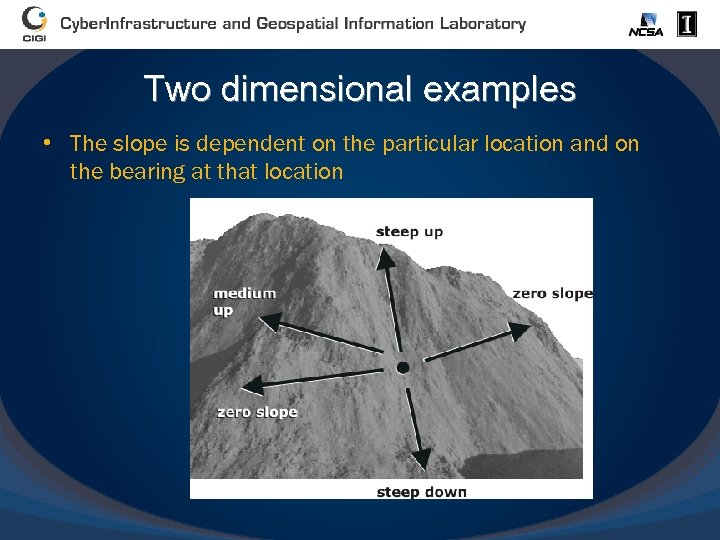Two dimensional examples • The slope is dependent on the particular location and on the bearing at that location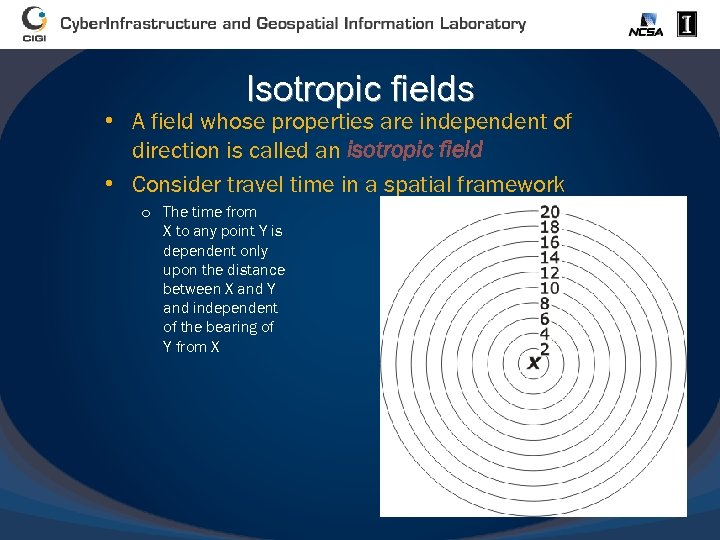Isotropic fields • A field whose properties are independent of direction is called an isotropic field • Consider travel time in a spatial framework o The time from X to any point Y is dependent only upon the distance between X and Y and independent of the bearing of Y from X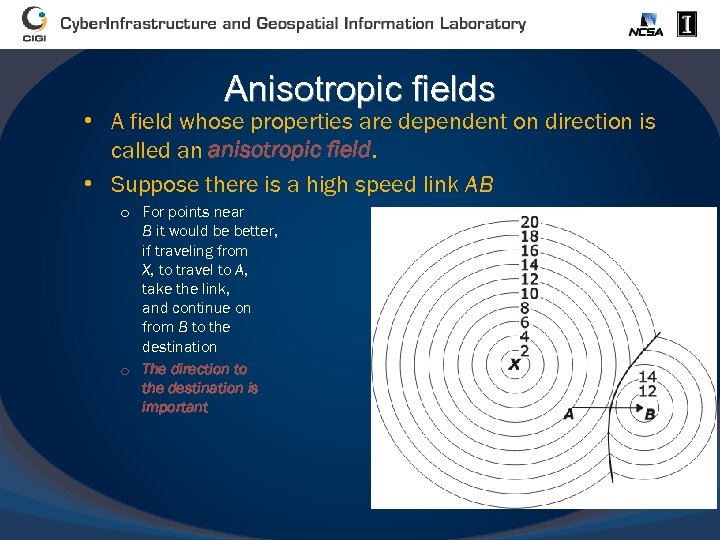Anisotropic fields • A field whose properties are dependent on direction is called an anisotropic field. • Suppose there is a high speed link AB o For points near B it would be better, if traveling from X, to travel to A, take the link, and continue on from B to the destination o The direction to the destination is important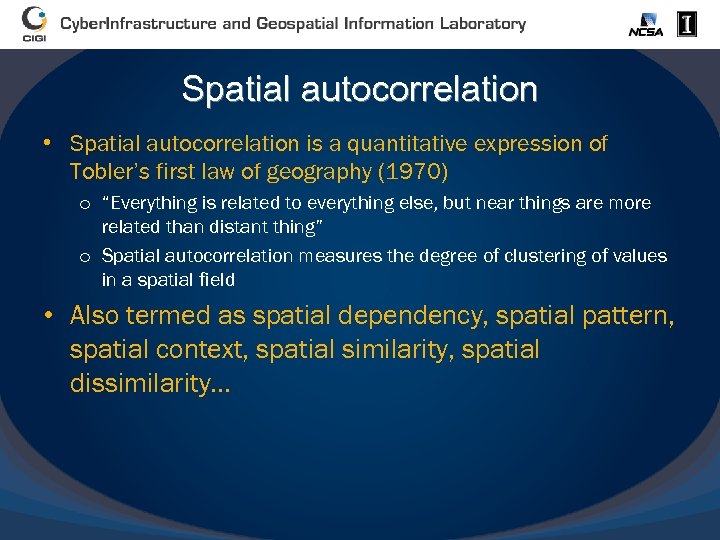Spatial autocorrelation • Spatial autocorrelation is a quantitative expression of Tobler’s first law of geography (1970) o “Everything is related to everything else, but near things are more related than distant thing” o Spatial autocorrelation measures the degree of clustering of values in a spatial field • Also termed as spatial dependency, spatial pattern, spatial context, spatial similarity, spatial dissimilarity…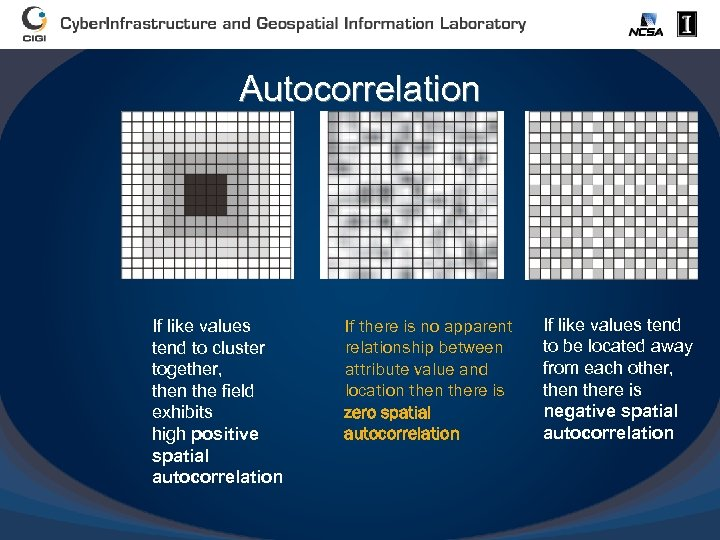Autocorrelation If like values tend to cluster together, then the field exhibits high positive spatial autocorrelation If there is no apparent relationship between attribute value and location there is zero spatial autocorrelation If like values tend to be located away from each other, then there is negative spatial autocorrelation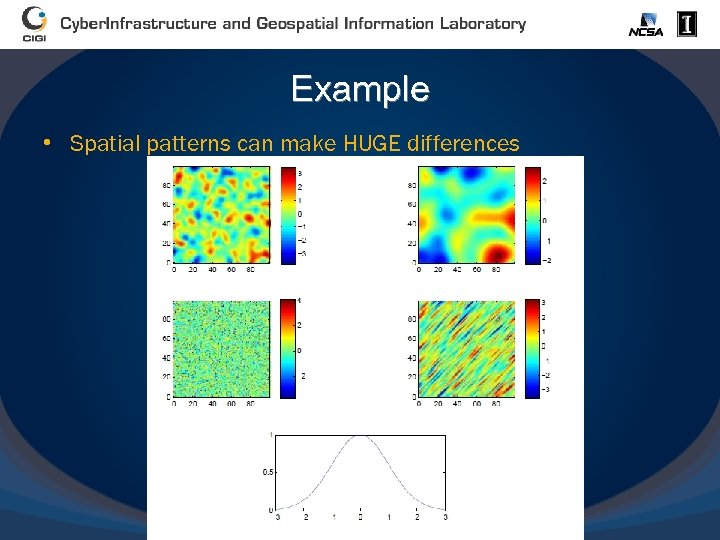Example • Spatial patterns can make HUGE differences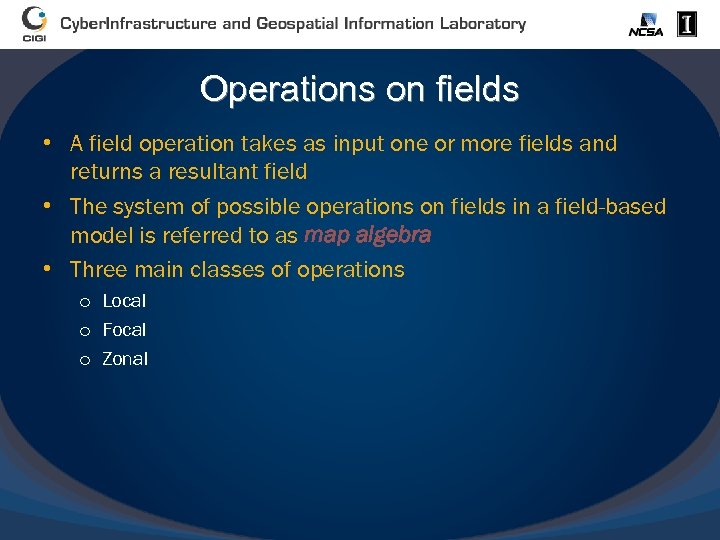Operations on fields • A field operation takes as input one or more fields and returns a resultant field • The system of possible operations on fields in a field-based model is referred to as map algebra • Three main classes of operations o Local o Focal o Zonal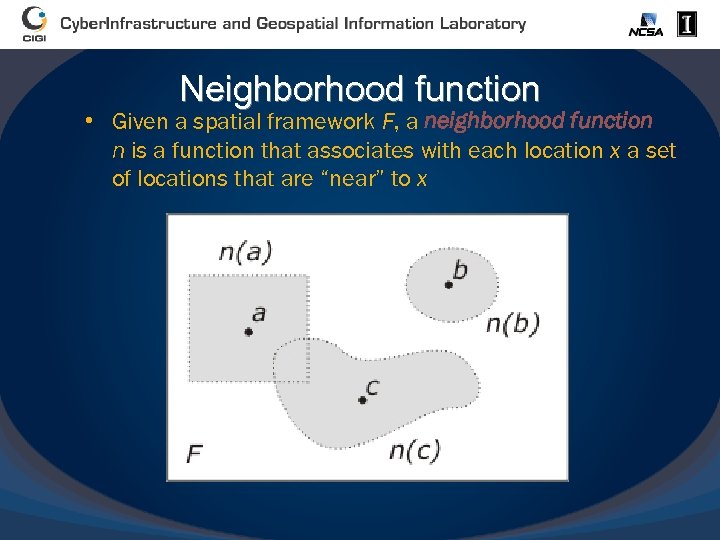Neighborhood function • Given a spatial framework F, a neighborhood function n is a function that associates with each location x a set of locations that are “near” to x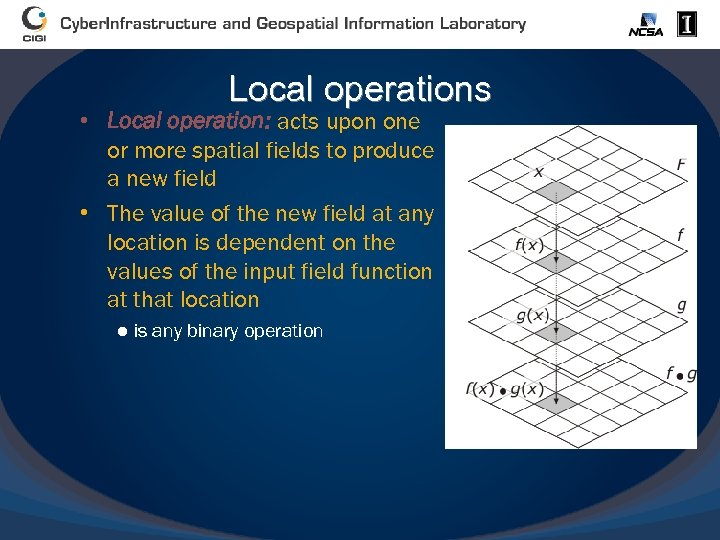Local operations • Local operation: acts upon one or more spatial fields to produce a new field • The value of the new field at any location is dependent on the values of the input field function at that location ● is any binary operation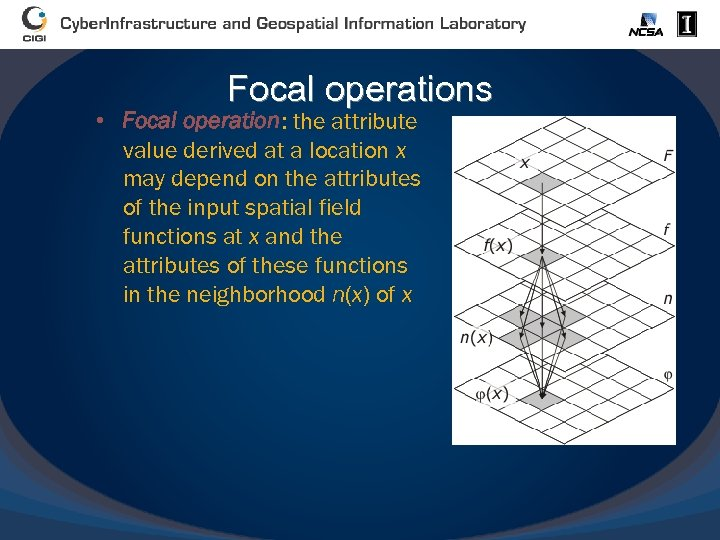Focal operations • Focal operation: the attribute value derived at a location x may depend on the attributes of the input spatial field functions at x and the attributes of these functions in the neighborhood n(x) of x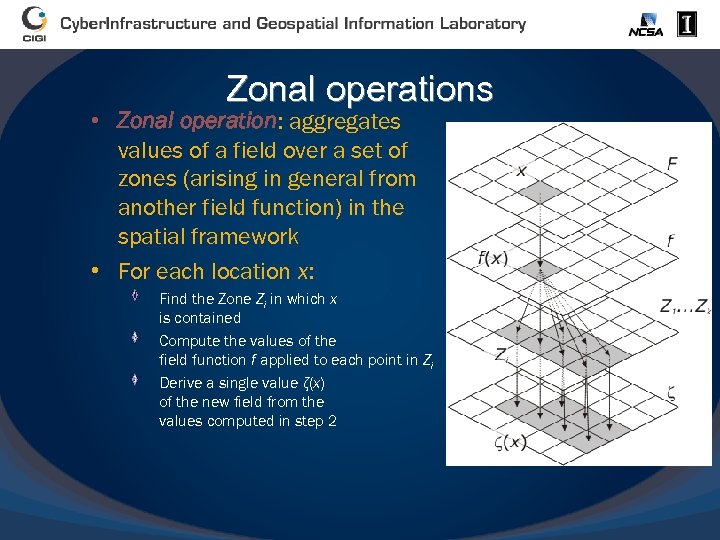Zonal operations • Zonal operation: aggregates values of a field over a set of zones (arising in general from another field function) in the spatial framework • For each location x: Find the Zone Zi in which x is contained Compute the values of the field function f applied to each point in Zi Derive a single value ζ(x) of the new field from the values computed in step 2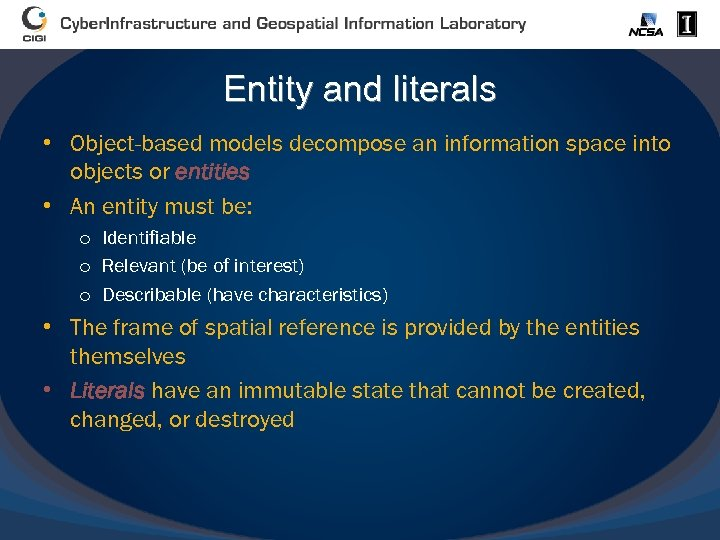Entity and literals • Object-based models decompose an information space into objects or entities • An entity must be: o Identifiable o Relevant (be of interest) o Describable (have characteristics) • The frame of spatial reference is provided by the entities themselves • Literals have an immutable state that cannot be created, changed, or destroyed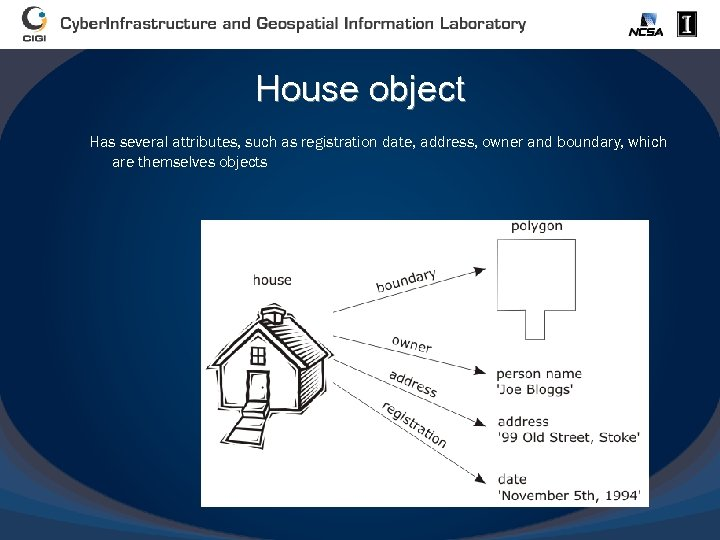House object Has several attributes, such as registration date, address, owner and boundary, which are themselves objects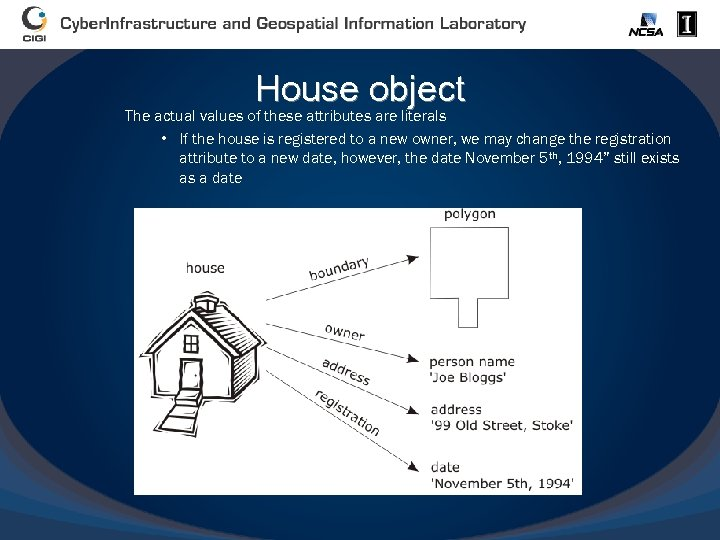House object The actual values of these attributes are literals • If the house is registered to a new owner, we may change the registration attribute to a new date, however, the date November 5 th, 1994” still exists as a date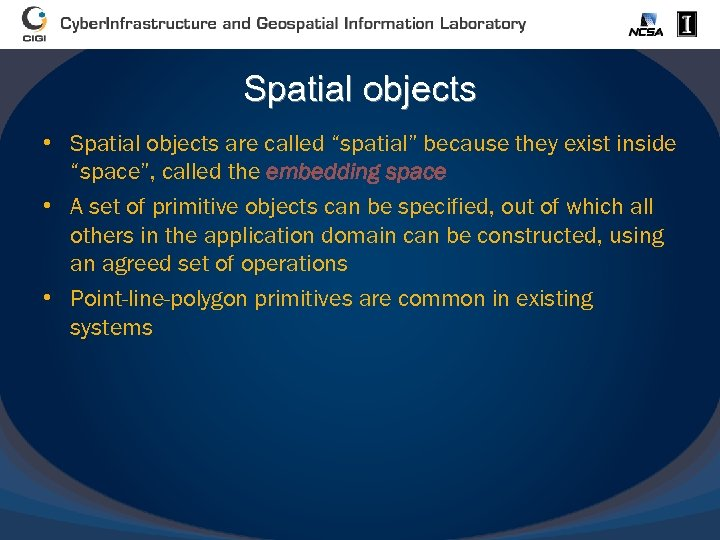Spatial objects • Spatial objects are called “spatial” because they exist inside “space”, called the embedding space • A set of primitive objects can be specified, out of which all others in the application domain can be constructed, using an agreed set of operations • Point-line-polygon primitives are common in existing systems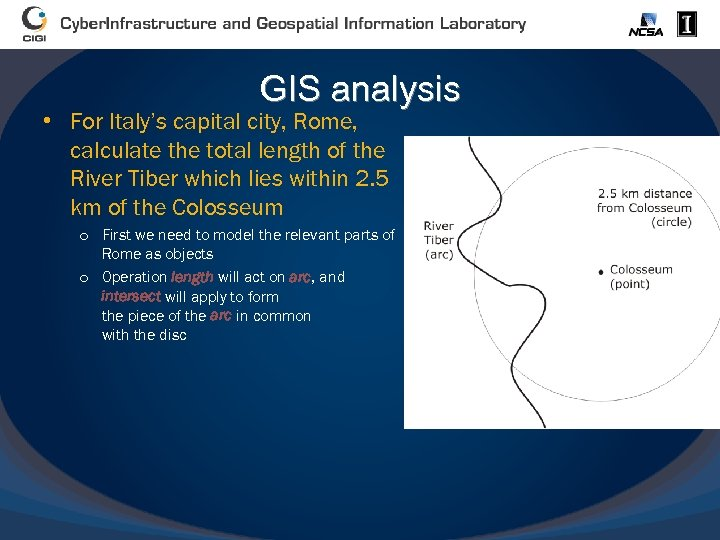GIS analysis • For Italy’s capital city, Rome, calculate the total length of the River Tiber which lies within 2. 5 km of the Colosseum o First we need to model the relevant parts of Rome as objects o Operation length will act on arc, and intersect will apply to form the piece of the arc in common with the disc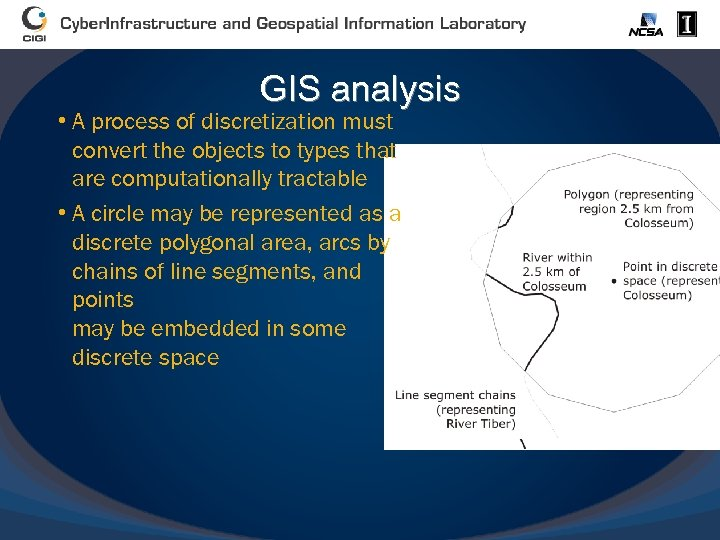GIS analysis • A process of discretization must convert the objects to types that are computationally tractable • A circle may be represented as a discrete polygonal area, arcs by chains of line segments, and points may be embedded in some discrete space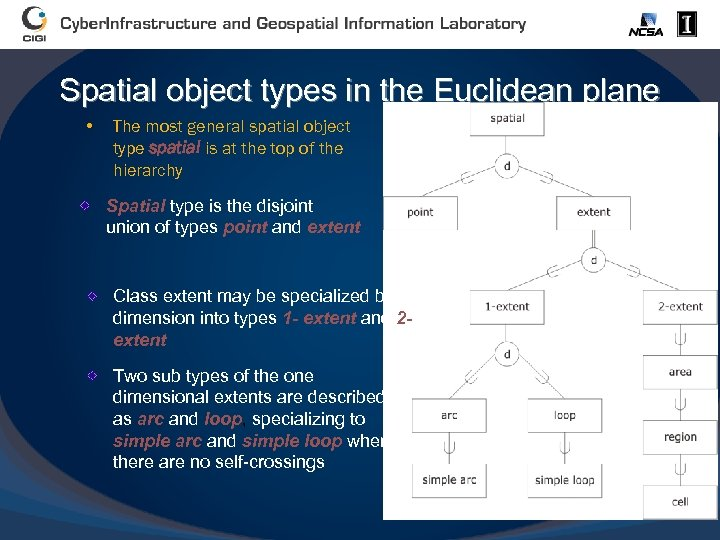Spatial object types in the Euclidean plane • The most general spatial object type spatial is at the top of the hierarchy Spatial type is the disjoint union of types point and extent Class extent may be specialized by dimension into types 1 - extent and 2 extent Two sub types of the one dimensional extents are described as arc and loop, specializing to simple arc and simple loop when there are no self-crossings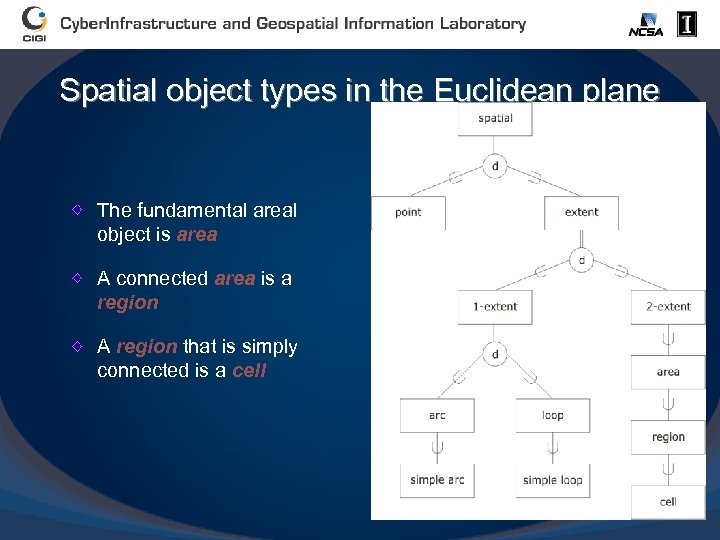Spatial object types in the Euclidean plane The fundamental areal object is area A connected area is a region A region that is simply connected is a cell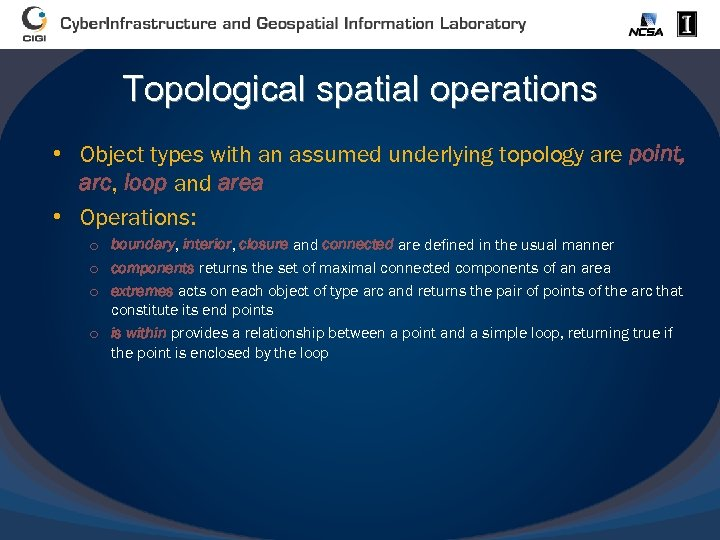Topological spatial operations • Object types with an assumed underlying topology are point, arc, loop and area • Operations: o boundary, interior, closure and connected are defined in the usual manner o components returns the set of maximal connected components of an area o extremes acts on each object of type arc and returns the pair of points of the arc that constitute its end points o is within provides a relationship between a point and a simple loop, returning true if the point is enclosed by the loop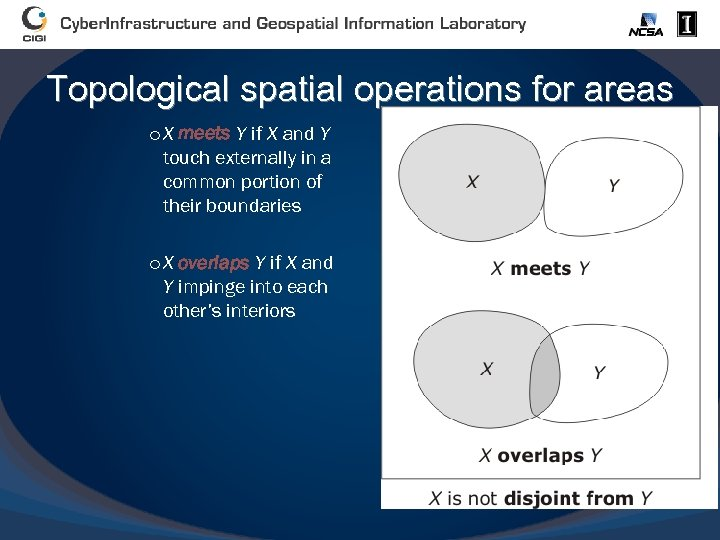Topological spatial operations for areas o X meets Y if X and Y touch externally in a common portion of their boundaries o X overlaps Y if X and Y impinge into each other’s interiors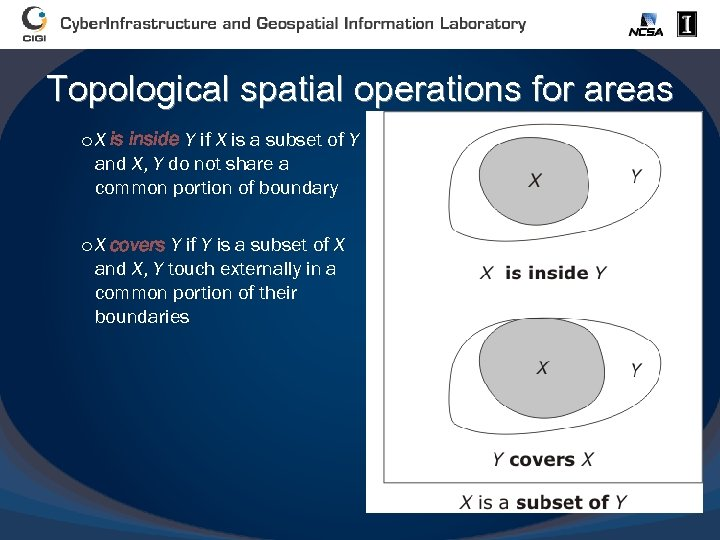Topological spatial operations for areas o X is inside Y if X is a subset of Y and X, Y do not share a common portion of boundary o X covers Y if Y is a subset of X and X, Y touch externally in a common portion of their boundaries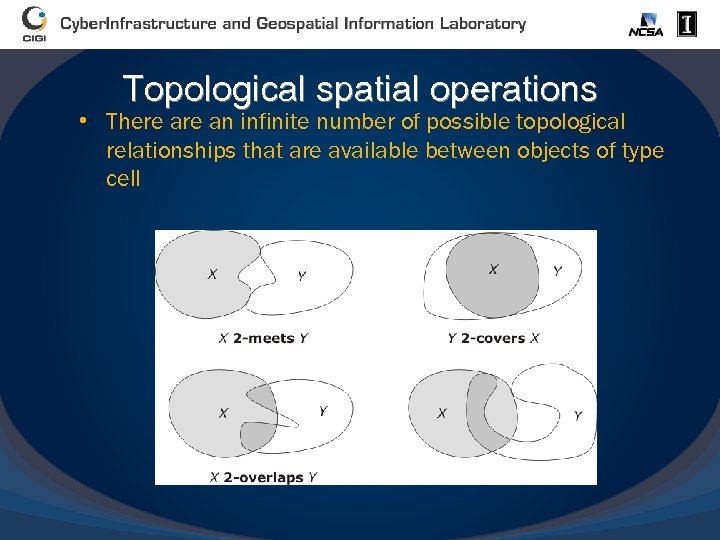Topological spatial operations • There an infinite number of possible topological relationships that are available between objects of type cell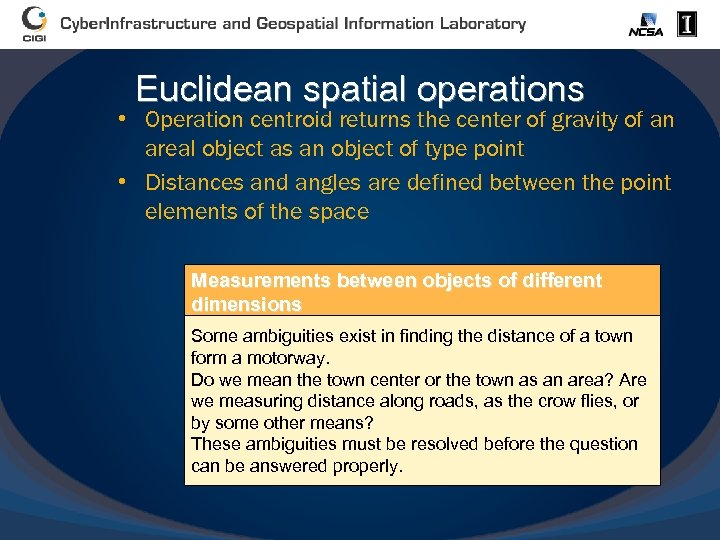Euclidean spatial operations • Operation centroid returns the center of gravity of an areal object as an object of type point • Distances and angles are defined between the point elements of the space Measurements between objects of different dimensions Some ambiguities exist in finding the distance of a town form a motorway. Do we mean the town center or the town as an area? Are we measuring distance along roads, as the crow flies, or by some other means? These ambiguities must be resolved before the question can be answered properly.Operations on spatial objects • All of these spatial operations can be thought of as operations on spatial literals. The operands are not affected by the application of the operation o For example, calculating the length of an arc cannot affect the arc itself • Another class of spatial operation acts upon spatially referenced objects, and alters the state of those objects o Create o Destroy o Update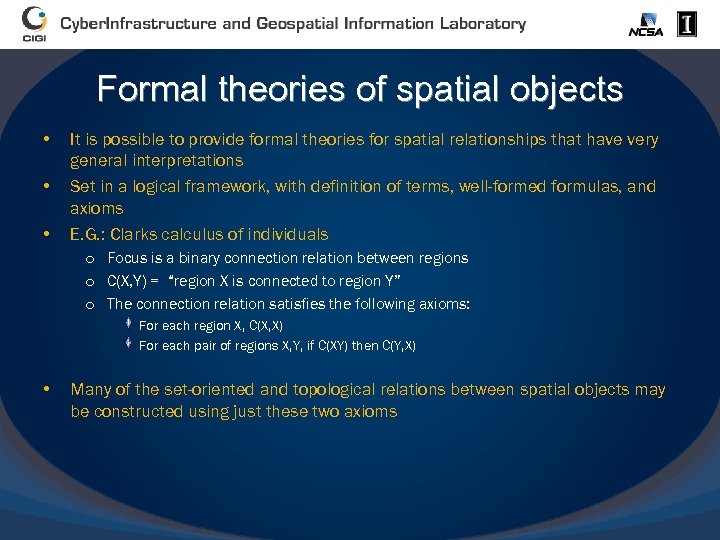Formal theories of spatial objects • • • It is possible to provide formal theories for spatial relationships that have very general interpretations Set in a logical framework, with definition of terms, well-formed formulas, and axioms E. G. : Clarks calculus of individuals o Focus is a binary connection relation between regions o C(X, Y) = “region X is connected to region Y” o The connection relation satisfies the following axioms: For each region X, C(X, X) For each pair of regions X, Y, if C(XY) then C(Y, X) • Many of the set-oriented and topological relations between spatial objects may be constructed using just these two axioms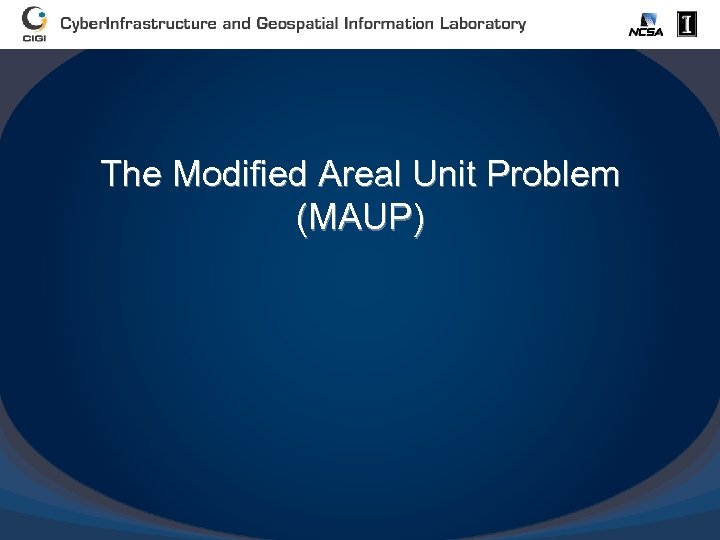The Modified Areal Unit Problem (MAUP)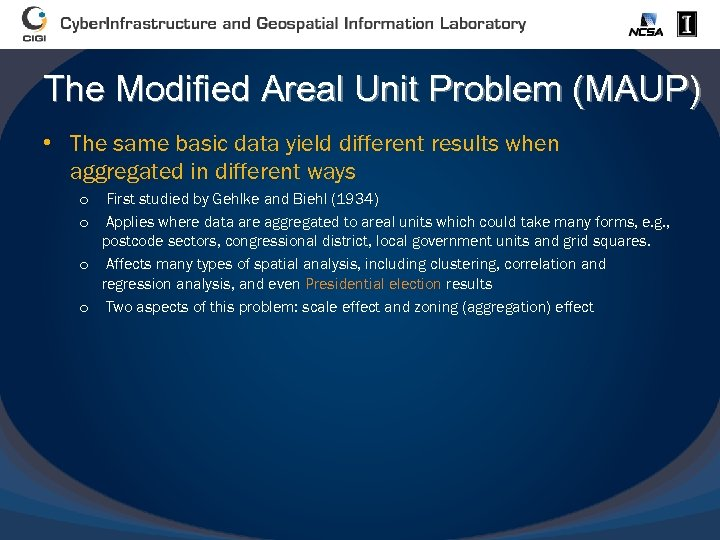The Modified Areal Unit Problem (MAUP) • The same basic data yield different results when aggregated in different ways First studied by Gehlke and Biehl (1934) Applies where data are aggregated to areal units which could take many forms, e. g. , postcode sectors, congressional district, local government units and grid squares. o Affects many types of spatial analysis, including clustering, correlation and regression analysis, and even Presidential election results o Two aspects of this problem: scale effect and zoning (aggregation) effect o o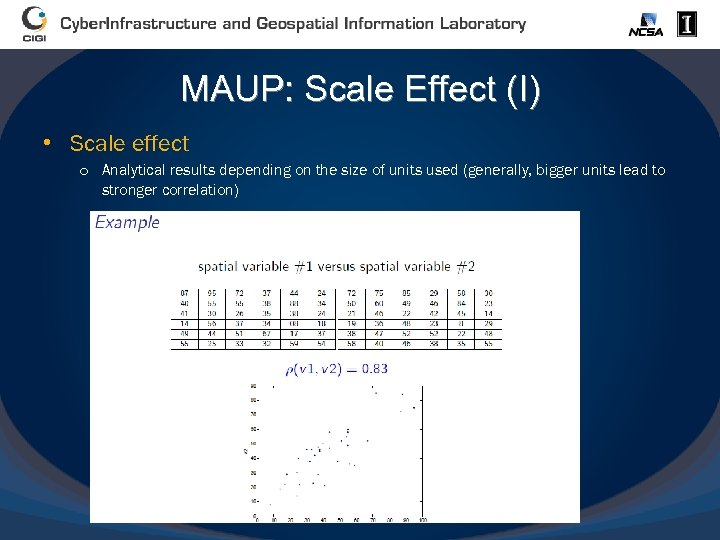MAUP: Scale Effect (I) • Scale effect o Analytical results depending on the size of units used (generally, bigger units lead to stronger correlation)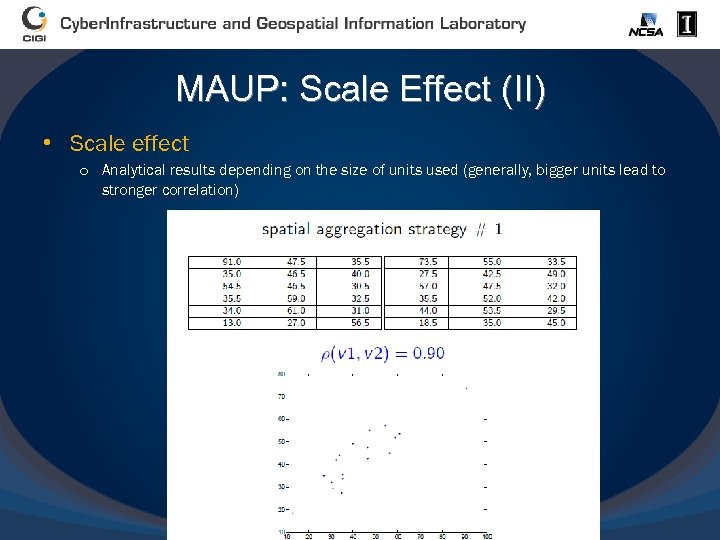MAUP: Scale Effect (II) • Scale effect o Analytical results depending on the size of units used (generally, bigger units lead to stronger correlation)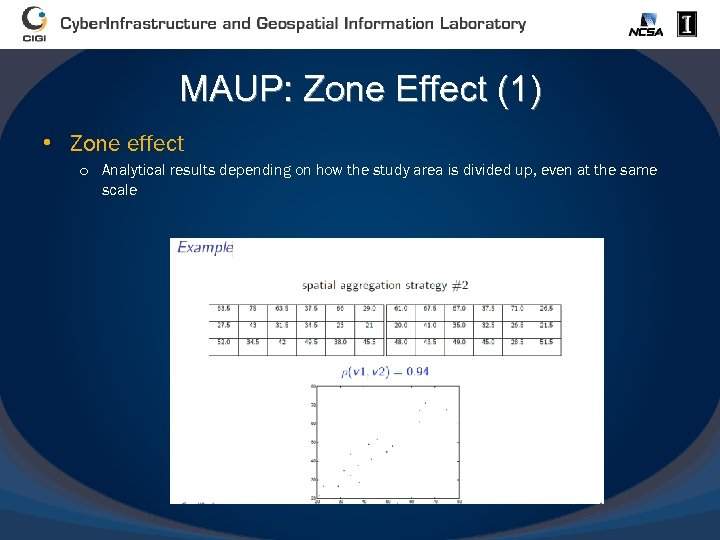MAUP: Zone Effect (1) • Zone effect o Analytical results depending on how the study area is divided up, even at the same scale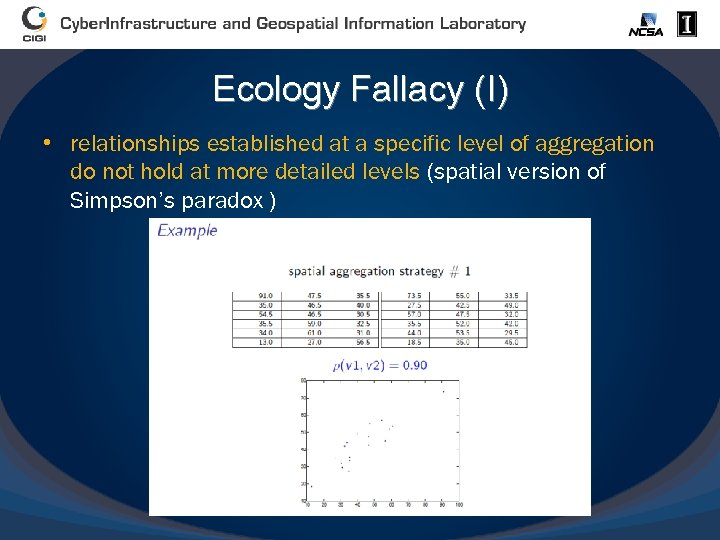Ecology Fallacy (I) • relationships established at a specific level of aggregation do not hold at more detailed levels (spatial version of Simpson’s paradox )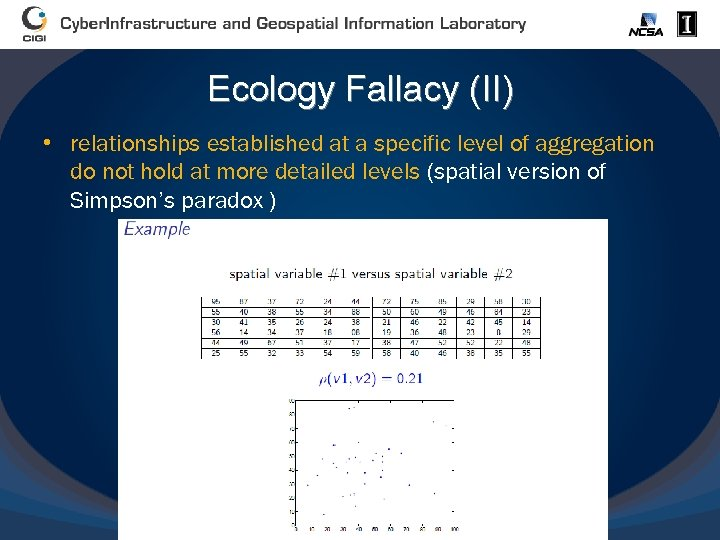Ecology Fallacy (II) • relationships established at a specific level of aggregation do not hold at more detailed levels (spatial version of Simpson’s paradox )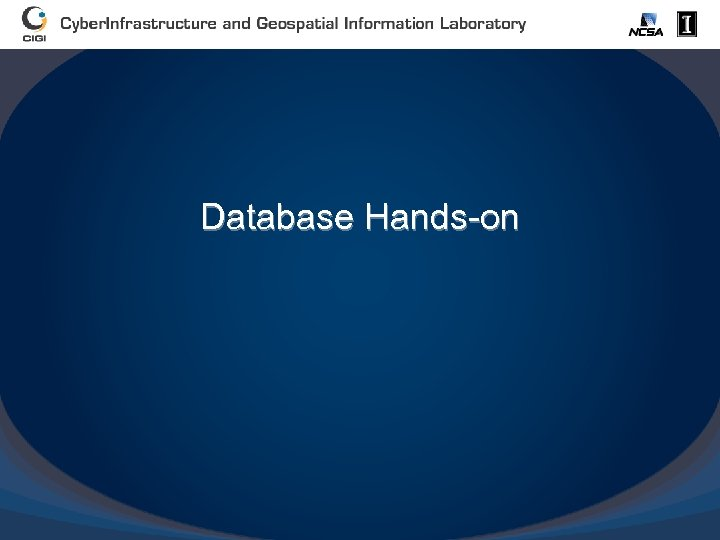Database Hands-on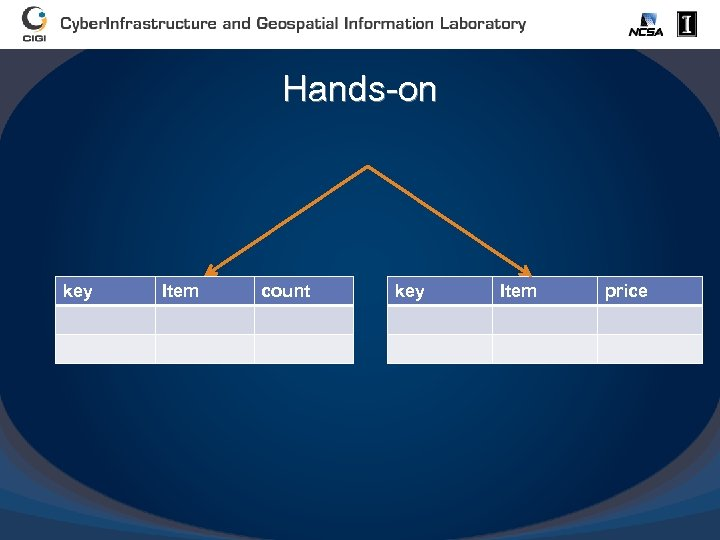Hands-on key Item count key Item price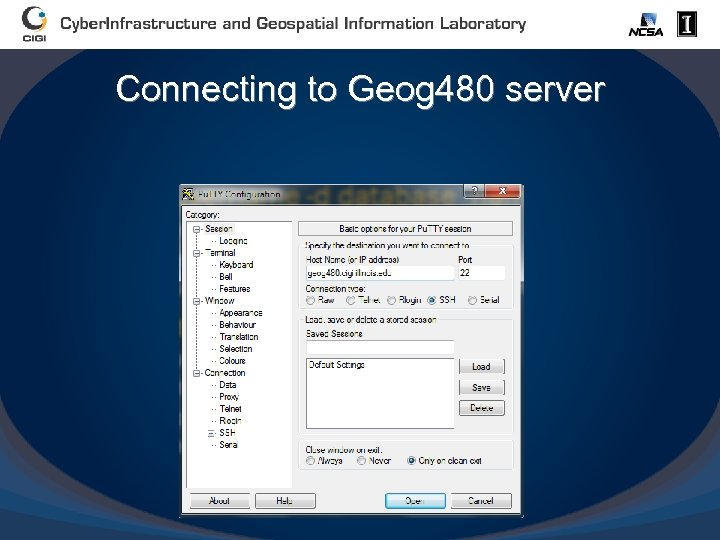Connecting to Geog 480 server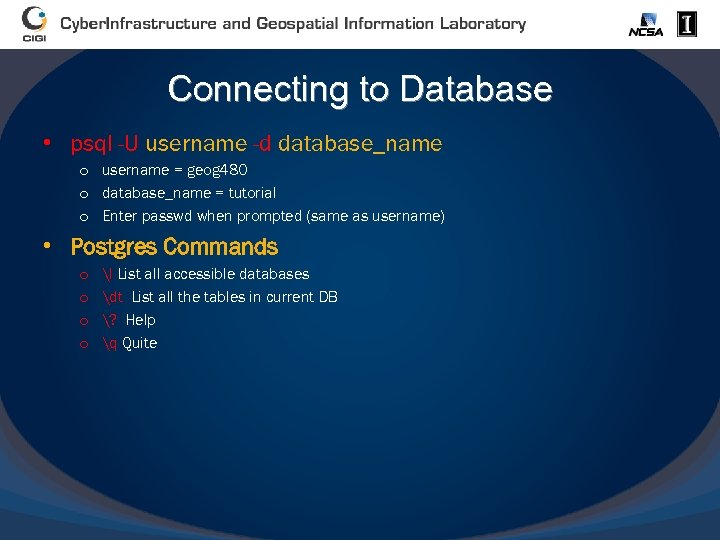Connecting to Database • psql -U username -d database_name o username = geog 480 o database_name = tutorial o Enter passwd when prompted (same as username) • Postgres Commands o o l List all accessible databases dt List all the tables in current DB ? Help q Quite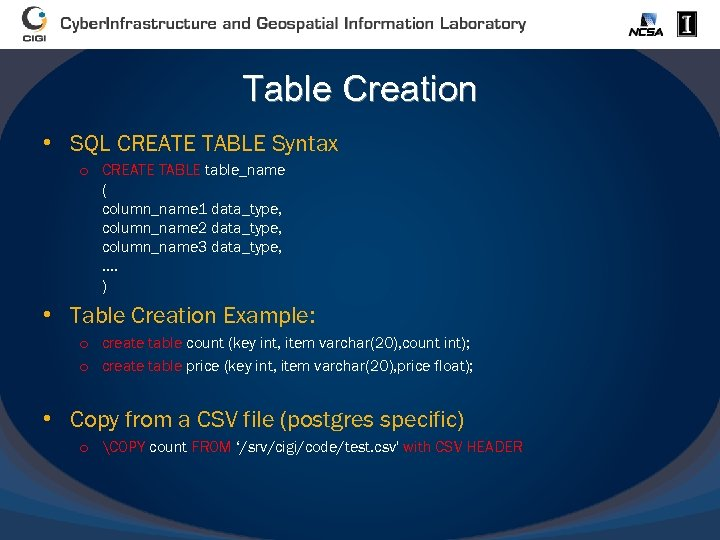Table Creation • SQL CREATE TABLE Syntax o CREATE TABLE table_name ( column_name 1 data_type, column_name 2 data_type, column_name 3 data_type, . . ) • Table Creation Example: o create table count (key int, item varchar(20), count int); o create table price (key int, item varchar(20), price float); • Copy from a CSV file (postgres specific) o COPY count FROM ‘/srv/cigi/code/test. csv' with CSV HEADER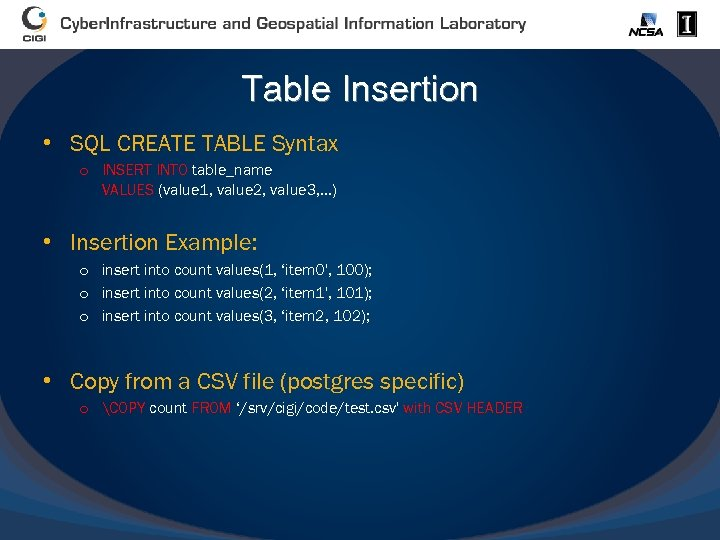Table Insertion • SQL CREATE TABLE Syntax o INSERT INTO table_name VALUES (value 1, value 2, value 3, . . . ) • Insertion Example: o insert into count values(1, ‘item 0', 100); o insert into count values(2, ‘item 1', 101); o insert into count values(3, ‘item 2, 102); • Copy from a CSV file (postgres specific) o COPY count FROM ‘/srv/cigi/code/test. csv' with CSV HEADER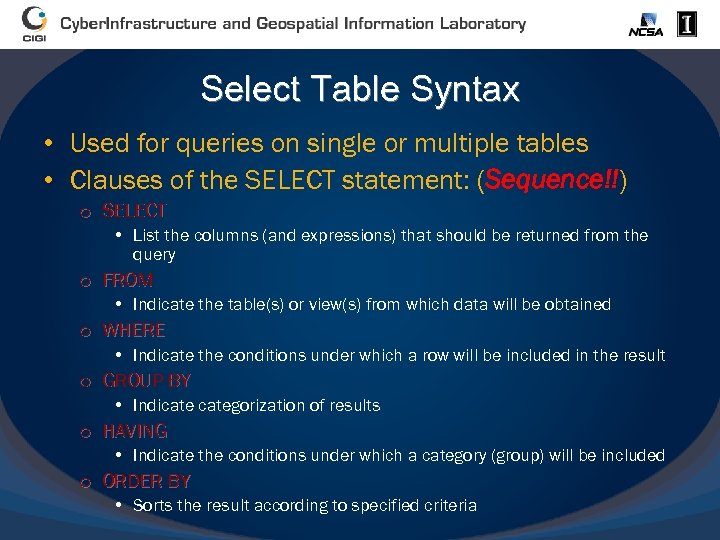Select Table Syntax • Used for queries on single or multiple tables • Clauses of the SELECT statement: (Sequence!!) o SELECT • List the columns (and expressions) that should be returned from the query o FROM • Indicate the table(s) or view(s) from which data will be obtained o WHERE • Indicate the conditions under which a row will be included in the result o GROUP BY • Indicategorization of results o HAVING • Indicate the conditions under which a category (group) will be included o ORDER BY • Sorts the result according to specified criteria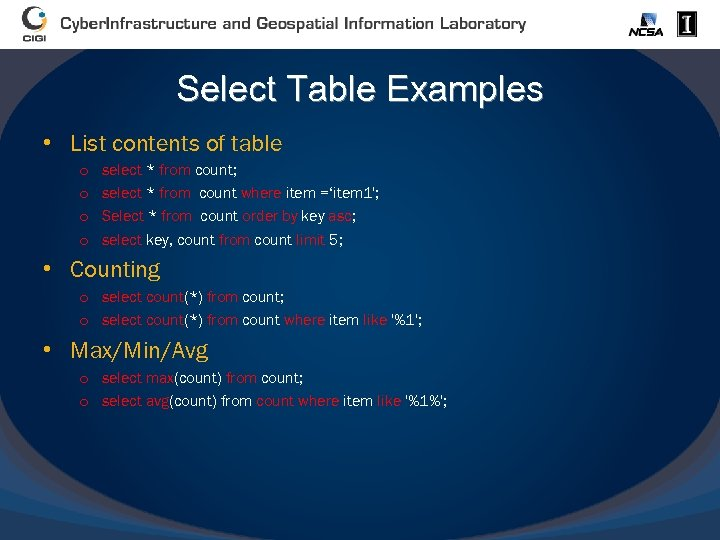Select Table Examples • List contents of table o o select * from count; select * from count where item =‘item 1'; Select * from count order by key asc; select key, count from count limit 5; • Counting o select count(*) from count; o select count(*) from count where item like '%1'; • Max/Min/Avg o select max(count) from count; o select avg(count) from count where item like '%1%';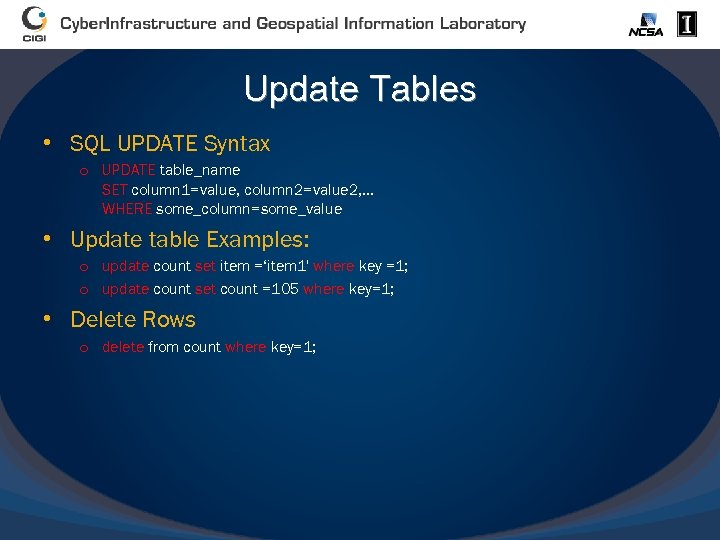Update Tables • SQL UPDATE Syntax o UPDATE table_name SET column 1=value, column 2=value 2, . . . WHERE some_column=some_value • Update table Examples: o update count set item =‘item 1' where key =1; o update count set count =105 where key=1; • Delete Rows o delete from count where key=1;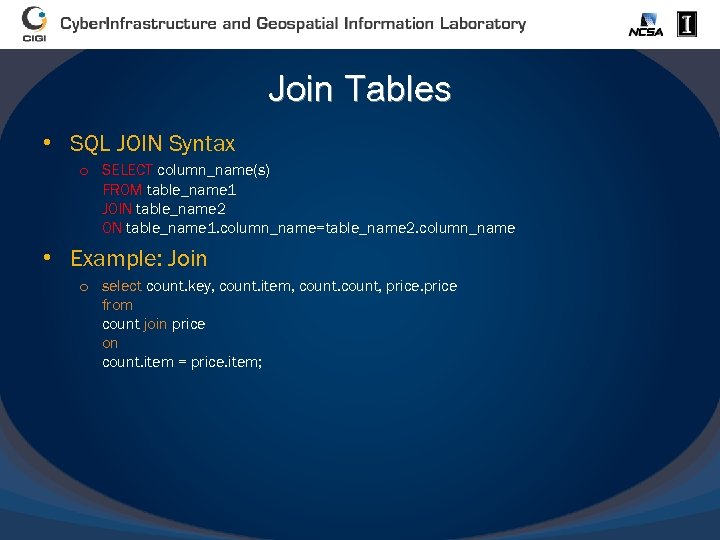Join Tables • SQL JOIN Syntax o SELECT column_name(s) FROM table_name 1 JOIN table_name 2 ON table_name 1. column_name=table_name 2. column_name • Example: Join o select count. key, count. item, count, price from count join price on count. item = price. item;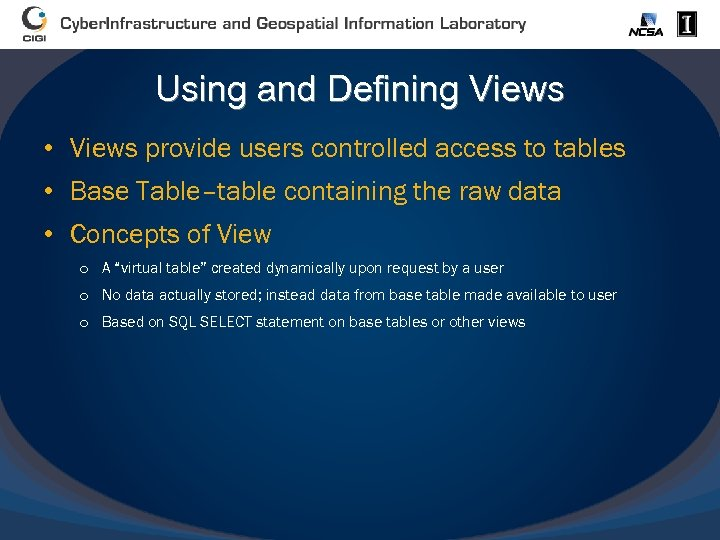Using and Defining Views • Views provide users controlled access to tables • Base Table–table containing the raw data • Concepts of View o A “virtual table” created dynamically upon request by a user o No data actually stored; instead data from base table made available to user o Based on SQL SELECT statement on base tables or other views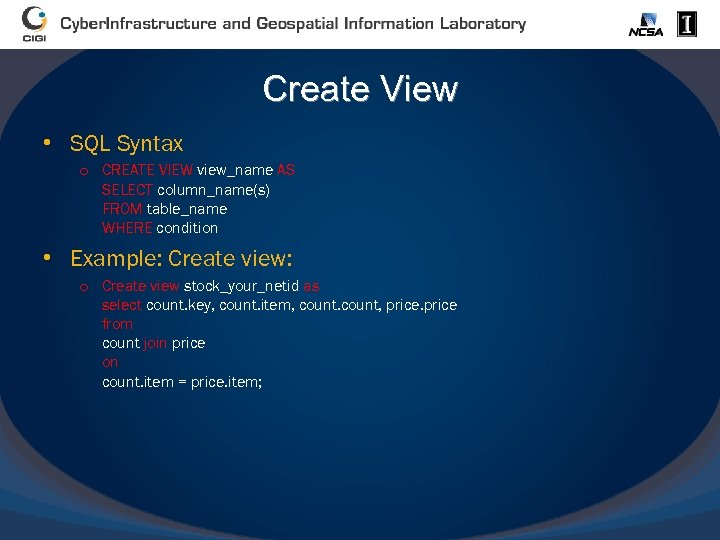Create View • SQL Syntax o CREATE VIEW view_name AS SELECT column_name(s) FROM table_name WHERE condition • Example: Create view: o Create view stock_your_netid as select count. key, count. item, count, price from count join price on count. item = price. item;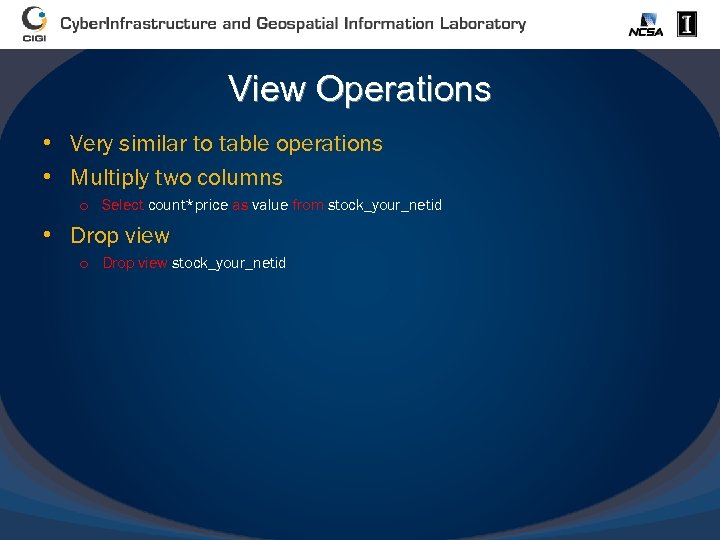View Operations • Very similar to table operations • Multiply two columns o Select count*price as value from stock_your_netid • Drop view o Drop view stock_your_netid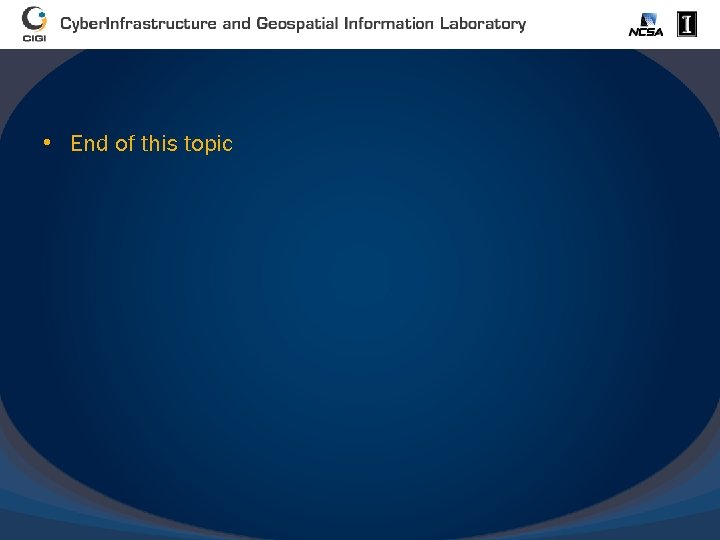• End of this topic